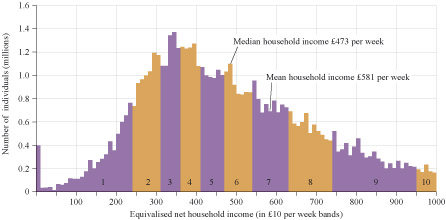Nature & Environment

### Become an OU studentEnvironment: treading lightly on the Earth

Start this free course now. Just create an account and sign in. Enrol and complete the course for a free statement of participation or digital badge if available.

# 2.1.1 Averages – mean and median

In this study note you will look at two different types of averages – the mean and the median.

## Study note: Averages mean and median

An average value is often used to provide a ‘typical’ value for a set of data. There are several ways of expressing a typical value, but the most common are the mean and the median.

Mean values

The mean value (commonly called the ‘average’, although it is just one type of average) is obtained by adding up all the values of a set of data and dividing by the number of items in the set. For example, if three individuals have carbon footprints of 13, 9 and 17 tonnes CO2e per year, their total footprint is 13 + 9 + 17 = 39 tonnes CO2e per year. Their mean footprint is the total divided by the number of individuals, i.e. 39/3 = 13 tonnes CO2e per person per year.

The mean is also used when several values contribute to an overall value, as in the case of assignment marks. If you had the assignment marks 67%, 82%, 45%, 75%, 77%, 68%, and they all counted equally towards your final score, you could work out your overall mean percentage score by adding the six scores together and dividing by that number of scores, i.e. (67 + 82 + 45 + 75 + 77 + 68)/6 = 69%.

To calculate the mean carbon footprint of a UK inhabitant (in 2013) using the information in Figure 4, you divide the total UK emissions of 1050 million tonnes CO2e by the 2013 UK population of 64.1 million. The result is 16.380655. Rounded to one decimal place this is 16.4 tonnes CO2e per person per year. (See Study Note: Rounding numbers, decimal places and significant figures at the end of this section.)

Median values

Although the example above gave a value for the amount of CO2e emissions that could be attributed to each person on an equal basis, this may not represent a ‘typical’ person. This is because there may be a group of heavy emitters that have a larger effect on the total (and hence the mean) than the majority of more modest emitters. In this case the median is a better indication of a typical individual’s footprint.

So, if the carbon footprints of a sample of 11 individuals were 21, 6, 15, 16, 10, 9, 13, 25, 12, 11, 27 tonnes CO2e per year, the mean footprint is 165/11 = 15 tonnes CO2e per year.

The median footprint is obtained by arranging the data into ascending order, as follows:

6, 9, 10, 11, 12, 13, 15, 16, 21, 25, 27 tonnes CO2e per year the median footprint is the middle value in the set = 13 tonnes CO2e per year, i.e. the sixth value in the set, with five below and five above it.

If there are an even number in the sample, say 10 individuals with footprints of

9, 10, 11, 12, 13, 15, 16, 21, 25, 27 tonnes CO2e per year

then the median is halfway between the two middle numbers (13 and 15), i.e. 14 tonnes CO2e per year, again with five values below and five above.

Consider another example, the UK’s income distribution. Some people have very high incomes and some have low incomes, with the majority spread between the extremes. Figure 6 shows the official distribution of the UK population by household incomes (after tax and before deduction of mortgages and rents) in 2014–15. The mean household income in 2014–15 is £581 per week (£30 212 per year), calculated by adding up all the incomes and dividing by the number of individuals living in households whose incomes were measured.

The median income is the amount which divides the income distribution into two equal groups, half having an income above that amount, and half having an income below that amount. The median household income in 2014–15 is £473 per week (£24 596 per year), which is less than the mean and more representative of an ‘average’ or ‘typical’ household.Figure 6 Distribution of the UK population by household incomes in 2014–15. Group 1 is the distribution of household incomes for the 10% of individuals living in the poorest households. Group 10 is the household incomes for the 10% of individuals living in the richest households (excluding 5.3 million individuals with a household income above £1000 per week). Equivalised means the incomes are adjusted to take account of the numbers of adults and children in a household (adapted from DWP, 2016).